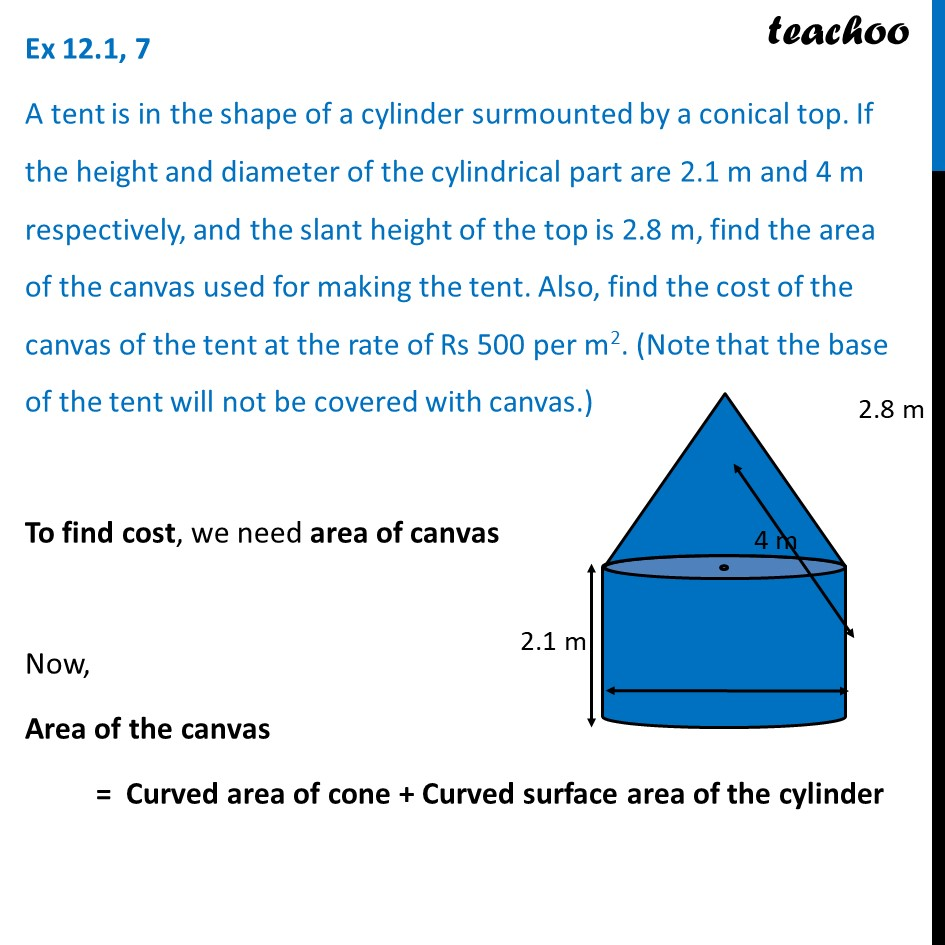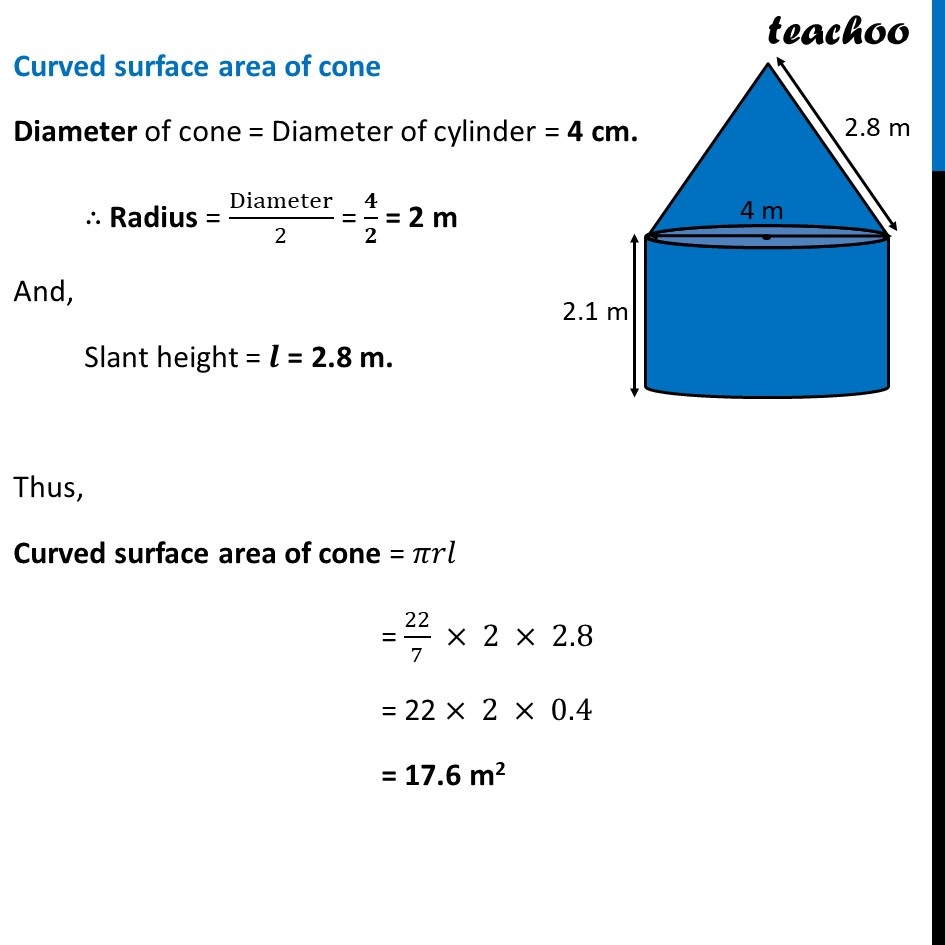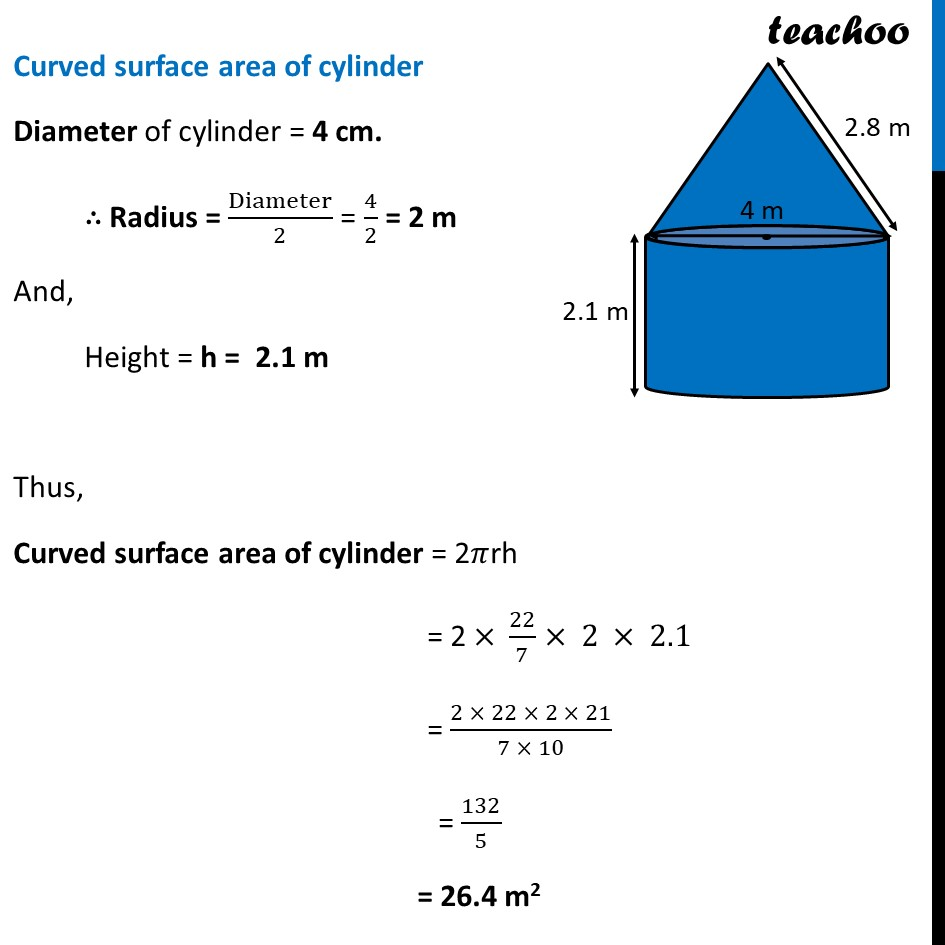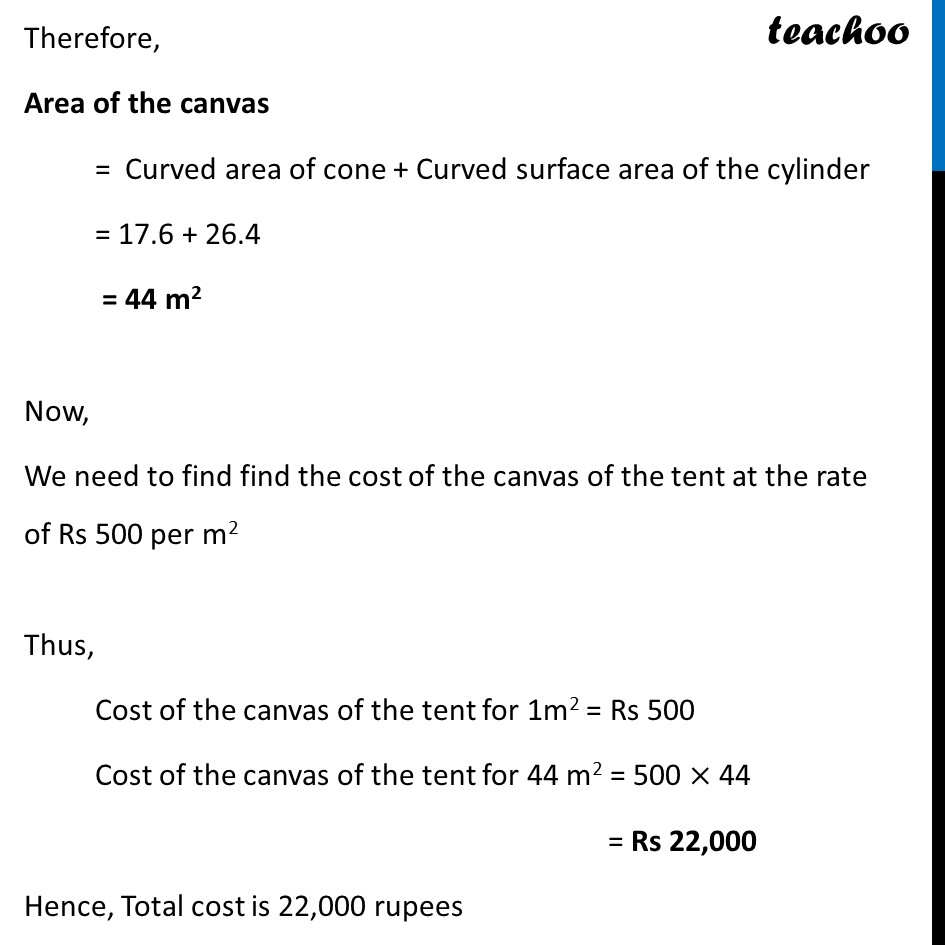Ex 12.1

Chapter 12 Class 10 Surface Areas and Volumes
Serial order wiseLearn in your speed, with individual attention - Teachoo Maths 1-on-1 Class

### Transcript

Ex 12.1, 7 A tent is in the shape of a cylinder surmounted by a conical top. If the height and diameter of the cylindrical part are 2.1 m and 4 m respectively, and the slant height of the top is 2.8 m, find the area of the canvas used for making the tent. Also, find the cost of the canvas of the tent at the rate of Rs 500 per m2. (Note that the base of the tent will not be covered with canvas.) To find cost, we need area of canvas Now, Area of the canvas = Curved area of cone + Curved surface area of the cylinder Curved surface area of cone Diameter of cone = Diameter of cylinder = 4 cm. ∴ Radius = Diameter/2 = 𝟒/𝟐 = 2 m And, Slant height = 𝒍 = 2.8 m. Thus, Curved surface area of cone = 𝜋𝑟𝑙 = 22/7 × 2 × 2.8 = 22 × 2 × 0.4 = 17.6 m2 Curved surface area of cylinder Diameter of cylinder = 4 cm. ∴ Radius = Diameter/2 = 4/2 = 2 m And, Height = h = 2.1 m Thus, Curved surface area of cylinder = 2𝜋rh = 2 × 22/7× 2 × 2.1 = (2 × 22 × 2 × 21)/(7 × 10) = 132/5 = 26.4 m2 Therefore, Area of the canvas = Curved area of cone + Curved surface area of the cylinder = 17.6 + 26.4 = 44 m2 Now, We need to find find the cost of the canvas of the tent at the rate of Rs 500 per m2 Thus, Cost of the canvas of the tent for 1m2 = Rs 500 Cost of the canvas of the tent for 44 m2 = 500 × 44 = Rs 22,000 Hence, Total cost is 22,000 rupees